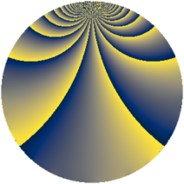# Properties

 Label 2850.2.xLevel $2850$ Weight $2$ Character orbit 2850.x Rep. character $\chi_{2850}(229,\cdot)$ Character field $\Q(\zeta_{10})$ Dimension $352$ Sturm bound $1200$

# Learn more about

## Defining parameters

 Level: $$N$$ $$=$$ $$2850 = 2 \cdot 3 \cdot 5^{2} \cdot 19$$ Weight: $$k$$ $$=$$ $$2$$ Character orbit: $$[\chi]$$ $$=$$ 2850.x (of order $$10$$ and degree $$4$$) Character conductor: $$\operatorname{cond}(\chi)$$ $$=$$ $$25$$ Character field: $$\Q(\zeta_{10})$$ Sturm bound: $$1200$$

## Dimensions

The following table gives the dimensions of various subspaces of $$M_{2}(2850, [\chi])$$.

Total New Old
Modular forms 2432 352 2080
Cusp forms 2368 352 2016
Eisenstein series 64 0 64

## Trace form

 $$352q + 88q^{4} - 4q^{5} + 4q^{6} + 88q^{9} + O(q^{10})$$ $$352q + 88q^{4} - 4q^{5} + 4q^{6} + 88q^{9} - 4q^{10} - 12q^{11} - 4q^{15} - 88q^{16} + 20q^{17} - 4q^{19} + 4q^{20} - 20q^{23} + 16q^{24} - 16q^{26} + 20q^{28} + 16q^{29} - 12q^{31} - 24q^{34} - 4q^{35} - 88q^{36} + 4q^{40} - 88q^{41} - 20q^{42} - 8q^{44} + 4q^{45} - 304q^{49} + 16q^{50} + 80q^{53} - 4q^{54} - 16q^{55} - 16q^{60} + 16q^{61} - 120q^{62} - 20q^{63} + 88q^{64} - 32q^{65} + 16q^{66} + 20q^{70} + 80q^{73} - 16q^{74} - 16q^{76} + 40q^{79} - 4q^{80} - 88q^{81} + 20q^{83} - 92q^{85} + 48q^{86} - 20q^{88} - 24q^{89} + 4q^{90} + 8q^{91} - 4q^{95} + 4q^{96} + 20q^{97} - 8q^{99} + O(q^{100})$$

## Decomposition of $$S_{2}^{\mathrm{new}}(2850, [\chi])$$ into newform subspaces

The newforms in this space have not yet been added to the LMFDB.

## Decomposition of $$S_{2}^{\mathrm{old}}(2850, [\chi])$$ into lower level spaces

$$S_{2}^{\mathrm{old}}(2850, [\chi]) \cong$$ $$S_{2}^{\mathrm{new}}(25, [\chi])$$$$^{\oplus 8}$$$$\oplus$$$$S_{2}^{\mathrm{new}}(50, [\chi])$$$$^{\oplus 4}$$$$\oplus$$$$S_{2}^{\mathrm{new}}(75, [\chi])$$$$^{\oplus 4}$$$$\oplus$$$$S_{2}^{\mathrm{new}}(150, [\chi])$$$$^{\oplus 2}$$$$\oplus$$$$S_{2}^{\mathrm{new}}(475, [\chi])$$$$^{\oplus 4}$$$$\oplus$$$$S_{2}^{\mathrm{new}}(950, [\chi])$$$$^{\oplus 2}$$$$\oplus$$$$S_{2}^{\mathrm{new}}(1425, [\chi])$$$$^{\oplus 2}$$